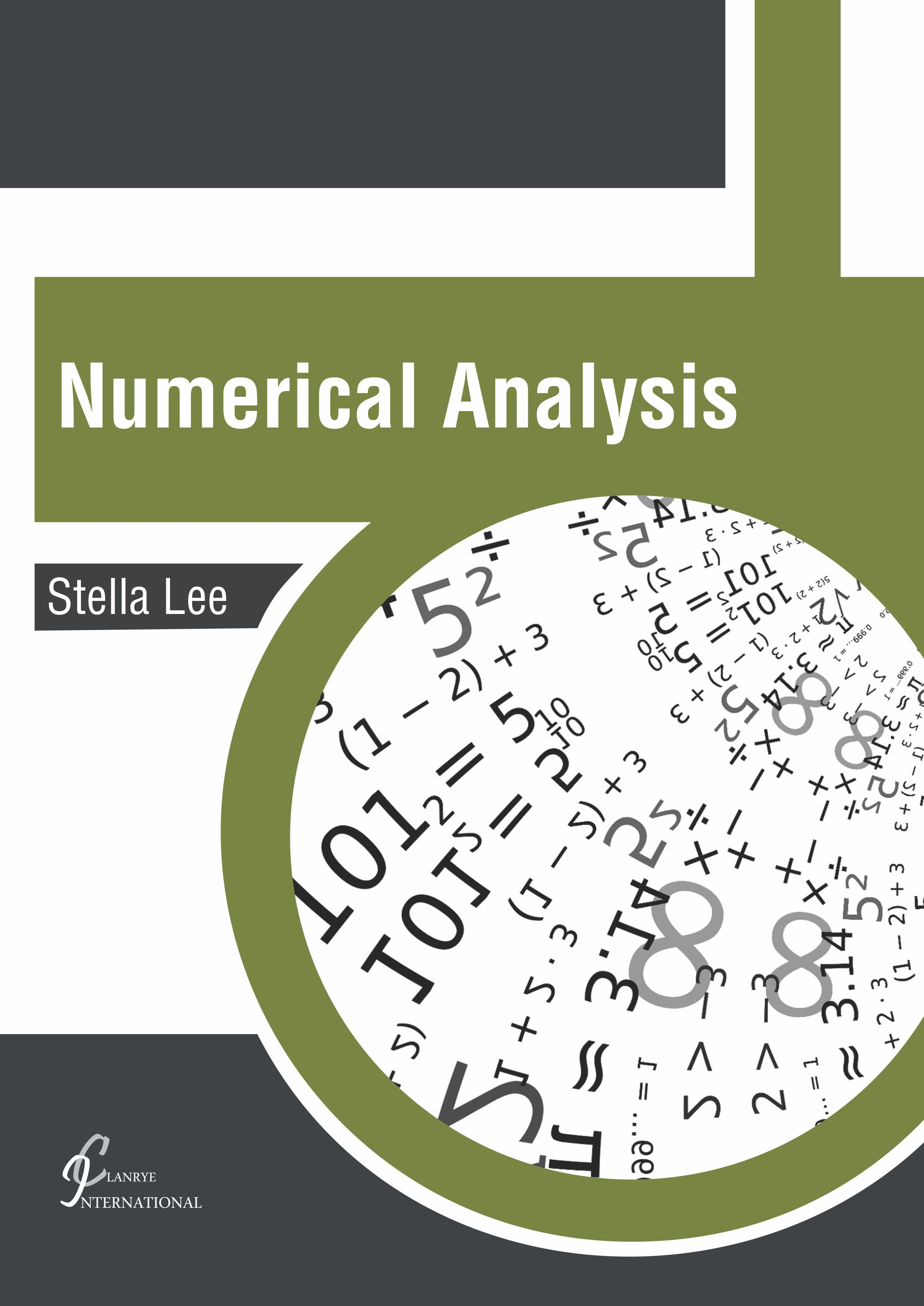# books

BROWSE BY

#### Author

Results 1 - 2 of 2Numerical Analysis Author : Stella Lee Subject : Numerical Analysis The study of algorithms that use numerical approximation for the problems of mathematical analysis is known as numerical analysis. Some of the major areas of study within this field are computing values Read MoreNumerical Analysis subtitle Author : Stella Lee Subject : Numerical Analysis The study of algorithms that use numerical approximation for the problems of mathematical analysis is known as numerical analysis. Some of the major areas of study within this field are computing values Read More
Results 1 - 2 of 2